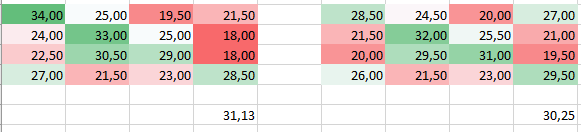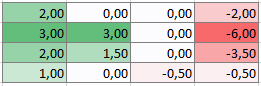# TDT confusing confusion matrixes

Dear All,

In my analysis I use 4 classes, I reach an accuracy of about 5% above chance (chance lvl- 25%). I tried to classify with the opposite order of the classes and it changes the outcome (the average is significantly different, matrixes for each person are different).
Do you have any idea what does it say about my outcome?Hi tryto,

I’m not sure what could have caused this behavior but it would be great if you could provide us with more detail about the analysis steps (e.g. what classifier, what steps for the confusion matrix or for accuracy etc.). Is this the average across subjects?

If you just take libsvm, then for multiclass problems remember that internally there will be several one-vs-one classifications happening. For 4 classes, there will be 6 pairwise classifications for one prediction. The majority vote usually wins. In case of ties in the decision, libsvm by default will always favor the first of these classes. We have incorporated a distance-based approach to avoid ties and such biases but I guess it can still happen depending on how you run your analysis.

Best,
Martin

EDIT: We used to have a distance-based approach in an alpha version but removed it to get the expected behavior by libsvm!

This classification has been done with all defaults (cfg = decoding_defaults();). Those two matrixes are the averages across 25 subjects, and down, there is an average accuracy for each classification.

My first thought was also that this is due to the fact that it favours the first class. Especially because when I subtracted the second matrix from the first one I saw that the first and second classes are higher and the last two lower.Do you think that subtracting the difference of those two versions of matrixes from the first one would give me values without the bias?

I think a (somewhat funny) workaround would be to run all possible combinations of label orders and average the results. This would distribute the bias equally and thus eliminate it.

I would probably go for all pairwise classifications instead, since multiclass classification is based on this. This is fine if you don’t want to build a real-world classifier but are interested in the representational content. Computationally speaking, the nice thing is that you get the decision values for all pairwise comparisons anyway. From that, libsvm let’s you construct all pairwise classification accuracies in a single step.

To this end, use
`cfg.results.output = {'accuracy_matrix_minus_chance'};`
which gives you the entire matrix (now chance level is 50% and it’s just the pairs of comparisons, with NaN on the diagonal)

or
`cfg.results.output = {'accuracy_pairwise_minus_chance'};`
which gives you the average of all cells.

Best,
Martin

Hey tryto,

in the most recent version of TDT (3.999E, see our webpage) I added a new output measure for exactly this purpose:
transres_confusion_matrix_plus_undecided.m
You can use it by setting

cfg.results.output = confusion_matrix_plus_undecided ';

See “>> help confusion_matrix_plus_undecided” and “>> edit demo3_1_simpletoydata_multiclass_confusion” for more.

Hope that helps? Best!
Kai

1 Like

Yes, the pairwise accuracy option is much more informative in this case. Thank you for this suggestion. I will definitely use that.

That is exactly what i need. Although I’ve been having some issues with using this option. What i’m getting is always an error:

Matrix dimensions must agree.

Error in transres_confusion_matrix_plus_undecided (line 142)
majority_pred_ind = repmat(all_ulabels’, size(dv, 1), 1) == repmat(majority_pred_class, 1, size(dv, 2));

Error in decoding_transform_results (line 260)
output = feval(fhandle,decoding_out,chancelevel,cfg,data);

Error in decoding_generate_output (line 35)
output = decoding_transform_results(curr_output,decoding_out,chancelevel,cfg,data);

Error in decoding (line 568)
results = decoding_generate_output(cfg,results,decoding_out,i_decoding,curr_decoding,current_data);

Error in digits_mvpa_basic (line 118)
results = decoding(cfg);